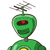# Find breadth of the rectangle whose length is 40cm and diagonal is 50 cm​

Find breadth of the rectangle whose length is 40cm and diagonal is 50 cm​

### 1 thought on “Find breadth of the rectangle whose length is 40cm and diagonal is 50 cm​”

1.$$by \: pythagoras \: theorem \\ = {a}^{2} + {b}^{2} = {c}^{2} \\ {40}^{2} + {b}^{2} = {50}^{2} \\ 1600 + {b}^{2} = 2500 \\ {b}^{2} = 2500 – 1600 \\ {b}^{2} = 900 \\ b = \sqrt{900} \\ b = 30$$